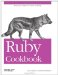# Building Queries Programmatically

#### Problem

You have to write fragments of SQL to pass parameters into an ActiveRecord query. Youd like to dispense with SQL altogether, and represent the query paramaters as a Ruby data structure.

#### Solution

Heres a simple solution. The method ActiveRecord::Base.find_by_map defined below picks up where find leaves off. Normally a query is represented by a SQL fragment, passed in as the :conditions argument. Here, the :conditions argument contains a mapping of database field names to the desired values:

```	require cookbook_dbconnect

class ActiveRecord::Base
def self.find_by_map(id, args={}.freeze)
sql = []
values = []
args[:conditions].each do |field, value|
sql << "#{field} = ?"
values << value
end if args[:conditions]
args[:conditions] = [sql.join( AND ), values]
find(id, args)
end
end
```

Heres find_by_map in action, using the BlogPost class first seen in Recipe 13.11:

```	activerecord_connect

class BlogPost < ActiveRecord::Base
end

BlogPost.create(:title => Game Review: Foosball Carnage,
:content => Four stars!)
BlogPost.create(:title => Movie Review: Foosball Carnage: The Movie,
:content => ero stars!)

BlogPost.find_by_map(:first,
:conditions => {:title =>
Game Review: Foosball Carnage }
).content
# => "Four stars!"
```

#### Discussion

ActiveRecord saves you from having to write a lot of SQL, but you still have to write out the equivalent of a SQL WHERE clause every time you call ActiveRecord::Base#find. The find_by_map method lets you define those queries as Ruby hashes.

But find_by_map only lets you run one type of query: the kind where you e restricting fields of the database to specific values. What if you want to do a query that matches a field with the LIKE construct, or combine multiple clauses into a single query with AND or OR?

A hash can only represent a very simple SQL query, but the Criteria object, below, can represent almost any WHERE clause. The implementation is more complex but the idea is the same. We define a data structure that can represent the WHERE clause of a SQL query, and a way of converting the data structure into a real WHERE clause.

Heres the basic class. A Criteria acts like a hash, except it maps a field name to a value and a SQL operator. Instead of mapping :title to Game Review: Foosball Carnage, you can map it to [%Foosball%, LIKE]. Each Criteria object can be chained to other objects as part of an AND or OR clause.

```	class Criteria < Hash
def initialize(values)
values.each { |k,v| add(k, *v) }
@or_criteria = nil
@and_criteria = nil
end

:private
attr_accessor :or_criteria, :and_criteria

:public
self[field] = [value, operation]
end

def or(criteria)
c = self
while c.or_criteria != nil
break if c == criteria
c = c.or_criteria
end

c.or_criteria = criteria
return self
end

def and(criteria)
c = self
while c.and_criteria != nil
break if c == criteria
c = c.and_criteria
end

c.and_criteria = criteria
return self
end
```

This method turns a Criteria object, and any other objects to which its chained, into a SQL string with substitutions, and an array of values to use in the substitutions:

```	class Criteria
def to_where_clause
sql = []
values = []
each do |field, value|
if value.respond_to? :to_str
value, operation = value, =
else
value, operation = value[0..1]
end
sql << "#{field} #{operation} ?"
values << value
end
sql = ( + sql.join( AND ) + )

if or_criteria
or_where = or_criteria.to_where_clause
sql = "(#{sql} OR #{or_where.shift})"
values += or_where
end

if and_criteria
and_where = and_criteria.to_where_clause
sql = "(#{sql} AND #{and_where.shift})"
values += and_where
end
return values.unshift(sql)
end
end
```

Now its simple to write a version of find that accepts a Criteria:

```	class ActiveRecord::Base
def self.find_by_criteria(id, criteria, args={}.freeze)
args = args.dup
args[:conditions] = criteria.to_where_clause
find(id, args)
end
end
```

Heres Criteria used to express a complex SQL WHERE clause with a little bit of Ruby code. This query searches the blog_post table for reviews of bad movies and good games. The movies and the games must not be about the game of cricket.

```	review = Criteria.new(:title => [\%Review%, LIKE])
bad_movie = Criteria.new(:title => ["%Movie%", LIKE],
:content => ero stars!)
good_game = Criteria.new(:title => [\%Game%, LIKE],
:content => Four stars!)
no_cricket = Criteria.new(:title => [\%Cricket%, NOT LIKE])

review.to_where_clause
# => ["((title LIKE ?) AND
# (((content = ? AND title LIKE ?) OR (content = ? AND title LIKE ?))
# AND (title NOT LIKE ?)))",
# "%Review%", "Zero stars!", "%Movie%", "Four stars!", "%Game%",
# "%Cricket%"]

BlogPost.find_by_criteria(:all, review).each { |post| puts post.title }
# Game Review: Foosball Carnage
# Movie Review: Foosball Carnage: The Movie
```

The technique is a general one. Its easier for a human to construct Ruby data structures than to write valid SQL clauses, so write code to convert the one into the other. You can use this technique wherever any library expects you to write SQL.

For instance, the find method expects SQL fragments representing a querys ORDER BY or GROUP BY clause. You could represent each as an array of fields, and generate the SQL as needed.

```	# Just an idea…
order_by = [[:title, ASC]]
```

• The Criteria class is inspired by the one in the Torque ORM library for Java (http://db.apache.org/torque/)Ruby Cookbook (Cookbooks (OReilly))
ISBN: 0596523696
EAN: 2147483647
Year: N/A
Pages: 399